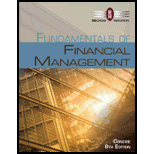# REAUZED RATES OF RETURN Stocks A and B have the following historical returns: Year Stock A’s Returns, r A Stock B’s Returns, r B 2009 (18.00%) (14.50%) 2010 33.00 21.80 2011 15.00 30.50 2012 (0.50) (7.60) 2013 27.00 26.30 a. Calculate the average rate of return for each stock during the period 2009 through 2013. b. Assume that someone held a portfolio consisting of 50% of Stock A and 50% of Stock B. What would the realized rate of return on the portfolio have been each year? What would the average return on the portfolio have been during this period? c. Calculate the standard deviation of returns for each stock and for the portfolio. d. Calculate the coefficient of variation for each stock and for the portfolio. e. Assuming you arc a risk-averse investor, would you prefer to hold Stock A, Stock B, or the portfolio? Why?### Fundamentals of Financial Manageme...

8th Edition
Eugene F. Brigham + 1 other
Publisher: Cengage Learning
ISBN: 9781285065137

#### Solutions

Chapter
Section### Fundamentals of Financial Manageme...

8th Edition
Eugene F. Brigham + 1 other
Publisher: Cengage Learning
ISBN: 9781285065137
Chapter 8, Problem 20P
Textbook Problem
130 views

## REAUZED RATES OF RETURN Stocks A and B have the following historical returns: Year Stock A’s Returns, rA Stock B’s Returns, rB 2009 (18.00%) (14.50%) 2010 33.00 21.80 2011 15.00 30.50 2012 (0.50) (7.60) 2013 27.00 26.30 a. Calculate the average rate of return for each stock during the period 2009 through 2013. b. Assume that someone held a portfolio consisting of 50% of Stock A and 50% of Stock B. What would the realized rate of return on the portfolio have been each year? What would the average return on the portfolio have been during this period? c. Calculate the standard deviation of returns for each stock and for the portfolio. d. Calculate the coefficient of variation for each stock and for the portfolio. e. Assuming you arc a risk-averse investor, would you prefer to hold Stock A, Stock B, or the portfolio? Why?

a.

Summary Introduction

To determine: The average rate of return for each stock during the given period.

Portfolio:

The portfolio refers to a group of financial assets like bonds, stocks, and equivalents of cash. The portfolio is held by investors and financial users. A portfolio is constructed based on the risk tolerance and the objectives of the company.

The Required Rate of Return:

The required rate of return is the rate, which should be minimum earned on an investment to keep that investment running in the market. When the required return is earned, only then the users and the companies invest in that particular investment.

### Explanation of Solution

Calculate the average rate of return for both of the stock.

The formula to calculate the average rate of return is,

Averagerateofreturn=SumofvalueofstocksNumberofstocks

Calculation of the average rate of return for stock A:

Substitute (18%), 33%, 15%, (0.5%) and 27% for value of stock A and 5 for the number of stocks in the above formula.

Averagerateofreturn=[(18%)+33%+15%+(0

b.

Summary Introduction

To determine: The realized rate of return on the portfolio for each year and the average return on portfolio for the given assumptions.

c.

Summary Introduction

To determine: The standard deviation of returns for each stock and for the portfolio.

d.

Summary Introduction

To determine: The coefficient of variation for each stock and for the portfolio.

The Coefficient of Variation:

The coefficient of variation is a tool to determine the risk. It determines the risk per unit of return. It is used for measurement when the expected returns are same for two data.

e.

Summary Introduction

To determine: The stock or the portfolio to be chosen and the reason for it.

### Still sussing out bartleby?

Check out a sample textbook solution.

See a sample solution

#### The Solution to Your Study Problems

Bartleby provides explanations to thousands of textbook problems written by our experts, many with advanced degrees!

Get Started

Find more solutions based on key concepts
IRR Refer to problem 11-1. What is the projects IRR?

Fundamentals of Financial Management (MindTap Course List)